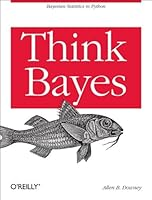# Think Bayes3 Reviews
2013-10-01
210 pages

## Book Description

If you know how to program with Python and also know a little about probability, you’re ready to tackle Bayesian . With this book, you'll learn how to solve statistical problems with Python instead of mathematical notation, and use discrete probability distributions instead of continuous mathematics. Once you get the math out of the way, the Bayesian fundamentals will become clearer, and you’ll begin to apply these techniques to real-world problems.

Bayesian statistical methods are becoming more common and more important, but not many resources are available to help beginners. Based on undergraduate classes taught by author Allen Downey, this book’s computational approach helps you get a solid start.

• Use your existing skills to learn and understand Bayesian statistics
• Work with problems involving estimation, prediction, decision analysis, evidence, and hypothesis
• Get started with simple examples, using coins, M&Ms, Dungeons & Dragons dice, paintball, and hockey
• Learn computational methods for solving real-world problems, such as interpreting scores, simulating kidney tumors, and the human microbiome.

Chapter 1. Bayes’s Theorem
Chapter 2. Computational Statistics
Chapter 3. Estimation
Chapter 4. More Estimation
Chapter 6. Decision Analysis
Chapter 7. Prediction
Chapter 8. Observer Bias
Chapter 9. Two Dimensions
Chapter 10. Approximate Bayesian Computation
Chapter 11. Hypothesis Testing
Chapter 12. Evidence
Chapter 13. Simulation
Chapter 14. A Hierarchical Model
Chapter 15. Dealing with Dimensions

## Book Details

• Title: Think Bayes
• Author:
• Length: 210 pages
• Edition: 1
• Language: English
• Publisher:
• Publication Date: 2013-10-01
• ISBN-10: 1449370780
• ISBN-13: 9781449370787